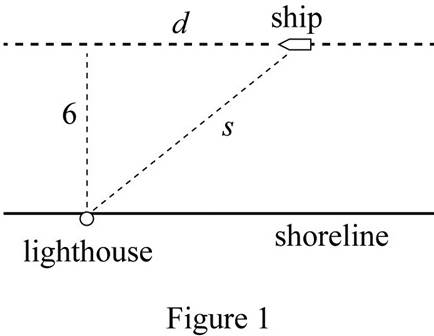# To express: The distance between the lighthouse and the ship as a function of d .### Single Variable Calculus: Concepts...

4th Edition
James Stewart
Publisher: Cengage Learning
ISBN: 9781337687805### Single Variable Calculus: Concepts...

4th Edition
James Stewart
Publisher: Cengage Learning
ISBN: 9781337687805

#### Solutions

Chapter 1.3, Problem 55E

(a)

To determine

## To express: The distance between the lighthouse and the ship as a function of d.

Expert Solution

The distance between the light house and the ship as of function d is, s=d2+36 .

### Explanation of Solution

Given:

The speed of the ship is 30 km/h.

The distance between the ship and shore is 6 km.

Diagram:

Draw a rough diagram to indicate the positions of the ship, lighthouse and shore as shown below in Figure 1.Consider the right triangle from Figure 1.

In order to find the distance s, use Pythogorean theorem.

s2=d2+62s=d2+36s=f(d)=d2+36

Therefore, the distance between the light house and the ship as a function d is, s=d2+36 .

(b)

To determine

### To express: The function d as a function of t where t is the time elapsed since noon.

Expert Solution

The function d as a function of t is, d=30t .

### Explanation of Solution

Given:

The speed of the ship is 30 km/h.

d is the distance in terms of kilometer and t is the time in hours.

Distance travelled is the product of speed and time.

Thus, the required function is, d=30t .

(c)

To determine

### To find: The composition function f∘g and explain.

Expert Solution

The composition function fg=625t2+1 and this function represents the distance between the light house and the ship since noon.

### Explanation of Solution

From part(a), s=f(d)=d2+36 and from part b, g(t)=d=30t .

The composition function is, fg=f(g(t)) .

Then f(g(t))=g(t)2+36

Substitute g(t) and find the value of the composition function.

f(30t)=(30t)2+36=900t2+36=36×25t2+36=625t2+1

Therefore, the composition function is, fg=625t2+1 and it represents the distance between the light house and the ship in terms of the elapsed time since noon.

### Have a homework question?

Subscribe to bartleby learn! Ask subject matter experts 30 homework questions each month. Plus, you’ll have access to millions of step-by-step textbook answers!